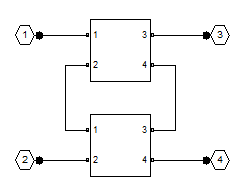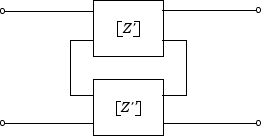rfckt.series

Series connected network

Description

Use the series class to represent networks of linear RF objects connected in series that are characterized by the components that make up the network. The following figure shows a pair of networks in a series configuration.Creation

Description

h = rfckt.series returns a series connected network object whose properties all have their default values.

example

h = rfckt.series('Ckts',value) returns a series connected network with elements specified in the name-value pair property Ckts.

Properties

expand all

Computed S-parameters, noise figure, OIP3, and group delay values, specified as rfdata.data object. Analyzed Result is a read-only property. For more information, see Algorithms.

Data Types: function_handle

Circuit objects in network, specified as a cell array of object handles. All circuits must be 2-port. By default, this property is empty.

Data Types: char

Object name, specified as a 1-by-N character array. Name is a read-only property.

Data Types: char

Number of ports, specified as a positive integer. nport is a read-only property. The default value is 2.

Data Types: double

Object Functions

 analyze Analyze RFCKT object in frequency domain calculate Calculate specified parameters for rfckt objects or rfdata objects circle Draw circles on Smith Chart extract Extract specified network parameters from rfckt object or data object listformat List valid formats for specified circuit object parameter listparam List valid parameters for specified circuit object loglog Plot specified circuit object parameters using log-log scale plot Plot circuit object parameters on X-Y plane plotyy Plot parameters of RF circuit or RF data on X-Y plane with two Y-axes getop Display operating conditions polar Plot specified object parameters on polar coordinates semilogx Plot RF circuit object parameters using log scale for x-axis semilogy Plot RF circuit object parameters using log scale for y-axis smith Plot circuit object parameters on Smith chart write Write RF data from circuit or data object to file getz0 Calculate characteristic impedance of RFCKT transmission line object read Read RF data from file to new or existing circuit or data object restore Restore data to original frequencies getop Display operating conditions groupdelay Group delay of S-parameter object or RF filter object or RF Toolbox circuit object

Examples

collapse all

Create a series connected RF network object using rfckt.series

tx1 = rfckt.txline;
tx2 = rfckt.txline;
ser = rfckt.series('Ckts',{tx1,tx2})
ser =
rfckt.series with properties:

Ckts: {[1x1 rfckt.txline]  [1x1 rfckt.txline]}
nPort: 2
AnalyzedResult: []
Name: 'Series Connected Network'

Algorithms

The analyze method computes the S-parameters of the AnalyzedResult property using the data stored in the Ckts property as follows:

1. The analyze method first calculates the impedance matrix of the series connected network. It starts by converting each component network's parameters to an impedance matrix. The following figure shows a series connected network consisting of two 2-port networks, each represented by its impedance matrix,where

$\begin{array}{l}\left[{Z}^{\prime }\right]=\left[\begin{array}{cc}{Z}_{11}{}^{\prime }& {Z}_{12}{}^{\prime }\\ {Z}_{21}{}^{\prime }& {Z}_{22}{}^{\prime }\end{array}\right]\\ \left[{Z}^{″}\right]=\left[\begin{array}{cc}{Z}_{11}{}^{\prime \text{​}\prime }& {Z}_{12}{}^{\prime \text{​}\prime }\\ {Z}_{21}{}^{\prime \text{​}\prime }& {Z}_{22}{}^{\prime \text{​}\prime }\end{array}\right]\end{array}$

2. The analyze method then calculates the impedance matrix for the series network by calculating the sum of the individual impedances. The following equation illustrates the calculations for two 2-port circuits.

$\left[Z\right]=\left[{Z}^{\prime }\right]+\left[{Z}^{″}\right]=\left[\begin{array}{cc}{Z}_{11}{}^{\prime }+{Z}_{11}{}^{\prime \text{​}\prime }& {Z}_{12}{}^{\prime }+{Z}_{12}{}^{\prime \text{​}\prime }\\ {Z}_{21}{}^{\prime }+{Z}_{21}{}^{\prime \text{​}\prime }& {Z}_{22}{}^{\prime }+{Z}_{22}{}^{\prime \text{​}\prime }\end{array}\right]$

3. Finally, analyze converts the impedance matrix of the series network to S-parameters at the frequencies specified in the analyze input argument freq.

 Ludwig, Reinhold and Pavel Bretchko, RF Circuit Design: Theory and Applications, Prentice-Hall, 2000.